# Family

94 boys are born per 100 girls. Determine the probability that there are two boys in a randomly selected family with three children.

Correct result:

p =  36.3054 %

#### Solution: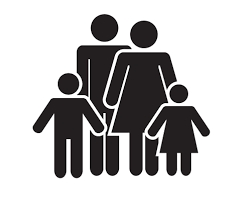We would be pleased if you find an error in the word problem, spelling mistakes, or inaccuracies and send it to us. Thank you!Tips to related online calculators
Looking for a statistical calculator?
Would you like to compute count of combinations?

## Next similar math problems:

• Boys and girlsThere are 11 boys and 18 girls in the classroom. Three pupils will answer. What is the probability that two boys will be among them?
• Component fail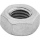There is a 90 percent chance that a particular type of component will perform adequately under high temperature conditions. If the device involved has four such components, determine the probability that the device is inoperable because exactly one of the
• Families 2There are 729 families having 6 children each. The probability of a girl is 1/3 and the probability of a boy is 2/3. Find the the number of families having 2 girls and 4 boys.
• RecordsRecords indicate 90% error-free. If 8 records are randomly selected, what is the probability that at least 2 records have no errors?
• Sick days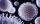In Canada, there are typically 261 working days per year. If there is a 4.9% chance that an employee takes a sick day. .. what is the probability an employee will use 17 OR MORE sick days in a year?
• FamilyWhat is the probability that a family with 7 childrens have: exactly 5 girls? 7 girls and 0 boys? Consider the birth probability of a girl is 48.69% and boy 51.31%.
• SalesFrom statistics of sales goods, item A buy 51% of people and item B buys 59% of people. What is the probability that from 10 people buy 2 item A and 8 item B?
• Internet anywhere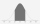In school, 60% of pupils have access to the internet at home. A group of 8 students is chosen at random. Find the probability that a) exactly 5 have access to the internet. b) At least 6 students have access to the internet
• All use computerIt is reported that 72% of working women use computers at work. Choose 3 women at random, find the probability that all 3 women use a computer in their jobs.
• TestThe teacher prepared a test with ten questions. The student has the option to choose one correct answer from the four (A, B, C, D). The student did not get a written exam at all. What is the probability that: a) He answers half correctly. b) He answers al
• Six questions testThere are six questions in the test. There are 3 answers to each - only one is correct. In order for a student to take the exam, at least four questions must be answered correctly. Alan didn't learn at all, so he circled the answers only by guessing. What
• Genetic diseaseOne genetic disease was tested positive in both parents of one family. It has been known that any child in this family has a 25% risk of inheriting the disease. A family has 3 children. What is the probability of this family having one child who inherited
• Probability of failures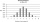In certain productions, the probability of failures is 0.01. Calculate the probability that there will be more than 1 failure among the 100 selected products if we return the selected products to the file after the check.
• Boys and girlsThere are eight boys and nine girls in the class. There were six children on the trip from this class. What is the probability that left a) only boys b) just two boys
• DiceWe throw five times the dice. What is the probability that six fits exactly twice?
• Playing cards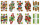From 32 playing cards containing 8 red cards, we choose 4 cards. What is the probability that just 2 will be red?
• Left handedIt is known that 25% of the population is left-handed. What is the probability that there is a maximum of three left-handers at a seminar where there are 30 participants?